Tamilnadu State Board New Syllabus Samacheer Kalvi 12th Maths Guide Pdf Chapter 10 Ordinary Differential Equations Ex 10.9 Textbook Questions and Answers, Notes.

## Tamilnadu Samacheer Kalvi 12th Maths Solutions Chapter 10 Ordinary Differential Equations Ex 10.9

Choose the most suitable answer from the given four alternatives:

Question 1.
The order and degree of the differential equation $$\frac { d^2y }{ dx^2 }$$ + ($$\frac { dy }{ dx }$$)1/3 + x1/4 = o are respectively
(a) 2, 3
(b) 3, 3
(c) 2, 6
(d) 2, 4
Solution:
(a) 2, 3
Hint: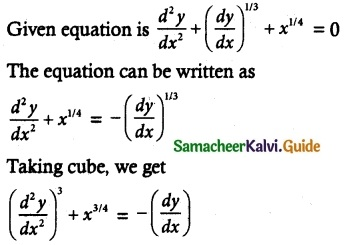In this equation, the highest order derivative is $$\frac { d^2y }{ dx^2 }$$ & its power is 3.
∴ Its order = 2 & degree = 3Question 2.
The differential equation representing the family of curves y = A cos (x + B), where A and B are parameters, is
(a) $$\frac { d^2y }{ dx^2 }$$ – y = 0
(b) $$\frac { d^2y }{ dx^2 }$$ + y = 0
(c) $$\frac { d^2y }{ dx^2 }$$ = 0
(d) $$\frac { d^2x }{ dy^2 }$$ = 0
Solution:
(b) $$\frac { d^2y }{ dx^2 }$$ + y = 0
Hint:
Given equation is y = A cos (x + B) …….. (1)
where A & B are parameters.
Differentiating equation (1) twice successively, because we have two arbitrary constants.
$$\frac { dy }{ dx }$$ = A sin (x + B)
Again differentiating $$\frac { d^2y }{ dx^2 }$$ = -A cos(x + B) = -y
∵ y = A cos (x + B
∵ $$\frac { d^2y }{ dx^2 }$$ + y = 0 as the required differential equation.

Question 3.
The order and degree of the differential equation, $$\sqrt { sin x }$$ (dx + dy) = $$\sqrt { cos x }$$ (dx – dy) is
(a) 1, 2
(b) 2, 2
(c) 1, 1
(d) 2, 1
Solution:
(c) 1, 1
Hint:
Given $$\sqrt { sin x }$$ (dx + dy) = $$\sqrt { cos x }$$ (dx – dy)
divide by dx on both sides, we get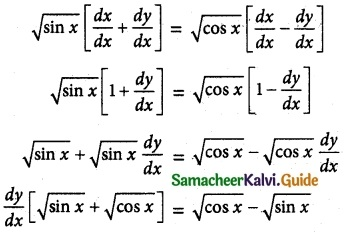In this equation, the highest, order derivative is $$\frac { dy }{ dx }$$ & its power is 1.
∴ Its order = 1 & degree = 1

Question 4.
The order of the differential equation of all circles with centre at (h, k) and radius ‘a’ is
(a) 2
(b) 3
(c) 4
(d) 1
Solution:
(a) 2
Hint:
We know that equation of a circle be (x – h)² + (y – k)² = a².
Here we have two constants. Therefore, Its order is 2.Question 5.
The differential equation of the family of curves y = Aex + Be-x, where A and B are arbitrary constants is
(a) $$\frac { d^2y }{ dx^2 }$$ + y = 0
(b) $$\frac { d^2y }{ dx^2 }$$ – y = 0
(c) $$\frac { dy }{ dx }$$ + y = 0
(d) $$\frac { dy }{ dx }$$ – y = 0
Solution:
(b) $$\frac { d^2y }{ dx^2 }$$ – y = 0
Hint:
Given y = Aex + Be-x ……… (1)
where A & B are arbitrary constants.
Differentiate equation(1) twice continuously, we get
(∵ Two constants so differentiate twice)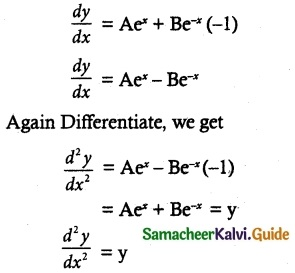$$\frac { d^2y }{ dx^2 }$$ – y = 0 as the required differential equation.

Question 6.
The general solution pf the differential equation $$\frac { dy }{ dx }$$ = $$\frac { y }{ x }$$ is
(a) xy = k
(b) y = k log x
(c) y = kx
(d) log y = kr
Solution:
(c) y = kx
Hint:
Given $$\frac { dy }{ dx }$$ = $$\frac { y }{ x }$$
The equation can be written as
$$\frac { dy }{ y }$$ = $$\frac { dx }{ x }$$
Integrating on both sides, we get
∫$$\frac { dy }{ y }$$ = ∫$$\frac { dy }{ x }$$
log y = log x + log k
log y = log k x [∵ log m + log n = log mn]
Remove log, we get
y = kx is a required differential equation

Question 7.
The solution of the differential equation 2x$$\frac { dy }{ dx }$$ – y = 3 represents
(a) straight lines
(b) circles
(c) parabola
(d) ellipse
Solution:
(c) parabola
Hint: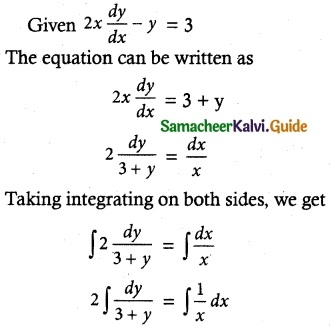2 log (3 + y) = log x + log k
log (3 + y)² = log kx
Remove log, we get
(3 + y)² = kx is a solution of the differential equation which is a Parabola.Question 8.
The solution of $$\frac { dy }{ dx }$$ + p(x) y = 0 is
(a) y = ce∫pdx
(b) y = ce-∫pdx
(c) x = ce-∫pdy
(d) x = ce∫pdy
Solution:
(b) y = ce-∫pdx
Hint:
Given $$\frac { dy }{ dx }$$ + p(x) y = 0
$$\frac { dy }{ dx }$$ = -p(x)y
The equation can be written as
$$\frac { dy }{ y }$$ = -p(x) dx
Taking integration on both sides, we get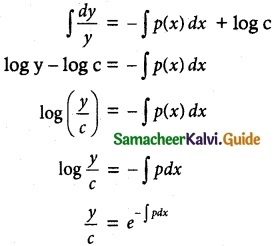∴ y = ce-∫pdx is a solution of the given differential equation.

Question 9.
The integrating factor of the differential equation $$\frac { dy }{ dx }$$ + y = $$\frac { 1+y }{ x }$$ is
(a) $$\frac { x }{ e^x }$$ + y = 0
(b) $$\frac { e^x }{ x }$$ – y = 0
(c) λex
(d) ex
Solution:
(b) $$\frac { e^x }{ x }$$ – y = 0
Hint:
Given differential equation is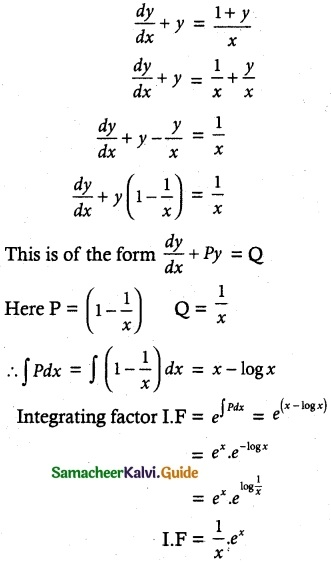Question 10.
The Integrating factor of the differential equation $$\frac { dy }{ dx }$$ + p(x) y = Q(x) is x, then p(x)
(a) x
(b) $$\frac { x^2 }{ 2 }$$
(c) $$\frac { 1 }{ x }$$
(d) $$\frac { 1 }{ x^2 }$$
Solution:
(c) $$\frac { 1 }{ x }$$
Hint:
The given differential equation is
$$\frac { dy }{ dx }$$ + p(x) y = Q(x)
Integrating factor e∫pdx = x
Taking log on both sides, we get ∫p dx = log x
Now differentiating, we get I
$$\frac { d }{ dx }$$ [∫p dx]= $$\frac { d }{ dx }$$ [log x]
∴ p = $$\frac { 1 }{ x }$$Question 11.
The degree of the differential equation
y(x) = 1 + $$\frac { dy }{ dx }$$ + $$\frac { 1 }{ 1.2 }$$ ($$\frac { dy }{ dx }$$)² + $$\frac { 1 }{ 1.2.3 }$$ ($$\frac { dy }{ dx }$$)³ + ……. is
(a) 2
(b) 3
(c) 1
(d) 4
Solution:
(c) 1
Hint:
Given
y(x) = 1 + $$\frac { dy }{ dx }$$ + $$\frac { 1 }{ 1.2 }$$ ($$\frac { dy }{ dx }$$)² + $$\frac { 1 }{ 1.2.3 }$$ ($$\frac { dy }{ dx }$$)³ + ……
Here the highest derivative term is $$\frac { dy }{ dx }$$ and its power is 1.
∴ Degree of the differential equation is 1.

Question 12.
If p and q are the order and degree of the differential equation y $$\frac { dy }{ dx }$$ +x³ ($$\frac { d^2y }{ dx^2 }$$) + xy = cos x, when
(a) p < q
(b) p = q
(c) p > q
(d) p exists and q does not exist
Solution:
(c) p > q
Hint:
Given equation is y$$\frac { dy }{ dx }$$ +x³ ($$\frac { d^2y }{ dx^2 }$$) + xy = cos x
The highest order derivative of the differential equation is $$\frac { d^2y }{ dx^2 }$$ and its degree is 1.
∴ Its order is p = 2 and degree q = 1
∴ p > q

Question 13.
The solution of the differential equation $$\frac { dy }{ dx }$$ + $$\frac { 1 }{ \sqrt{1-x^2} }$$ = 0 is
(a) y + sin-1 x = c
(b) x + sin-1 y = 0
(c) y² + 2sin-1 x = c
(d) x² + 2sin-1 y = 0
Solution:
(a) y + sin-1 x = c
Hint:
The given equation is $$\frac { dy }{ dx }$$ = –$$\frac { 1 }{ \sqrt{1-x^2} }$$
The equation can be written as
dy = $$\frac { 1 }{ \sqrt{1-x^2} }$$
Integrating on both sides, we get
∫dy = -∫$$\frac { dx }{ \sqrt{1-x^2} }$$
y = -sin-1 (x) + C
y + sin-1 (x) = C
∴ y + sin-1 (x) = C is a solution of the given differential equation.Question 14.
The solution of the differential equation $$\frac { dy }{ dx }$$ = 2xy is
(a) y = Ce
(b) y = 2x² + C
(c) y = Ce-x² + C
(d) y = x² + C
Solution:
(a) y = Ce
Hint:
Given
$$\frac { dy }{ dx }$$ = 2xy
The equation can be written as
$$\frac { dy }{ y }$$ = 2xdx
Taking integration on both sides, we get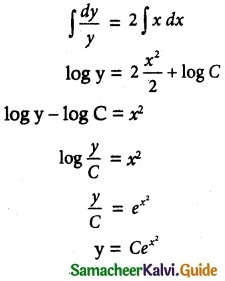∴ y = Ce is a solution to the differential equation.

Question 15.
The general solution of the differential equation log($$\frac { dy }{ dx }$$) = x + y is
(a) ex + ey = C
(b) ex + e-y = C
(c) e-x + ey = C
(d) e-x + e-y = C
Solution:
(b) ex + e-y = C
Hint:
Given differential equation is log $$\frac { dy }{ dx }$$ = x + y,
log $$\frac { dy }{ dx }$$ = x + y
$$\frac { dy }{ dx }$$ = ex+y = ex ey
$$\frac { dy }{ dx }$$ = ex ey
The given equation can be written as
$$\frac { dy }{ dx }$$ = ex ey
e-y dy = ex dx
Taking integration on both sides, we get
∫ e-y dy = ∫ ex dx
$$\frac { e^{-y} }{ -1 }$$ = ex + C
-e-y = ex + C
-ex – e-y = C
-(ex + e-y) = C
ex + e-y = -C
∴ ex + e-y = C Where – C = C
∴ ex + e-y = C is a solution of the given differential equation.

Question 16.
The solution of $$\frac { dy }{ dx }$$ = 2y-x is
(a) 2x + 2y = C
(b) 2x – 2y = C
(c) $$\frac { 1 }{ 2^x }$$ – $$\frac { 1 }{ 2^y }$$ = C
(d) x + y = C
Solution:
(c) $$\frac { 1 }{ 2^x }$$ – $$\frac { 1 }{ 2^y }$$ = C
Hint:
Given
$$\frac { dy }{ dx }$$ = 2y-x
The equation can be written as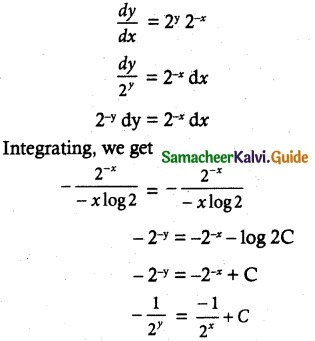$$\frac { 1 }{ 2^x }$$ – $$\frac { 1 }{ 2^y }$$ = C is a solution of the given differential equation.Question 17.
The solution of the differential equation
$$\frac { dy }{ dx }$$ = $$\frac { y }{ x }$$ + $$\frac { ∅(\frac { y }{ x }) }{ ∅(\frac { y }{ x }) }$$ is
(a) x∅($$\frac { y }{ x }$$) = k
(b) ∅($$\frac { y }{ x }$$) = kx
(c) y∅($$\frac { y }{ x }$$) = k
(d) ∅($$\frac { y }{ x }$$) = ky
Solution:
(b) ∅$$\frac { y }{ x }$$ = kx
Hint: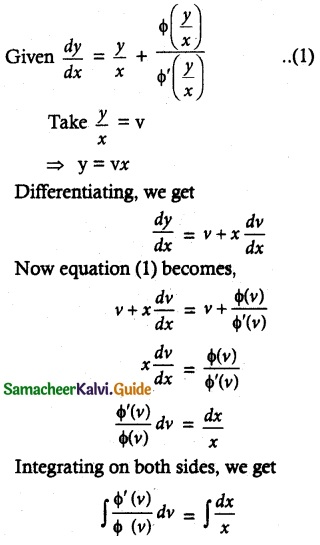log ∅(v) = log x + log k
log ∅(v) = log xk
∅(v) = kx
∅(y/x) = kx

Question 18.
If sin x is the integrating factor of the linear differential equation $$\frac { dy }{ dx }$$ + Py = Q, then P is
(a) log sin x
(b) cos x
(c) tan x
(d) cot x
Solution:
(d) cot x
Hint:
Given integrating factor
e∫pdx = sin x
∫pdx = log sin x ……. (1)
Differential equation (1) with respect to x, we get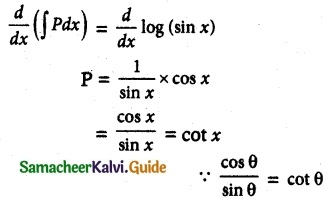∴ The value of P is cot x

Question 19.
The number of arbitrary constants in the general solutions of order n and n + 1 is respectively.
(a) n – 1, n
(b) n, n + 1
(c) n + 1, n + 2
(d) n + 1, n
Solution:
(b) n, n + 1
Hint:
If one arbitrary constant, Differentiate one time, so order is 1.
If two arbitrary constants, Differentiate two times, so order is 2.
.
.
.
If n arbitrary constants, Differentiate n times, so the order is n.
If n + 1 arbitrary constants, Differentiate n + 1 times, so order is n + 1.Question 20.
The number of arbitrary constants in the particular solution of a differential equation of third order is
(a) 3
(b) 2
(c) 1
(d) 0
Solution:
(d) 0

Question 21.
Integrating factor of the differential equation
$$\frac { dy }{ dx }$$ = $$\frac { x+y+1 }{ x+1 }$$ is
(a) $$\frac { 1 }{ x+1 }$$
(b) x+ 1
(c) $$\frac { 1 }{ \sqrt{x+1} }$$
(d) $$\sqrt { x+1 }$$
Solution:
(a) $$\frac { 1 }{ x+1 }$$
Hint: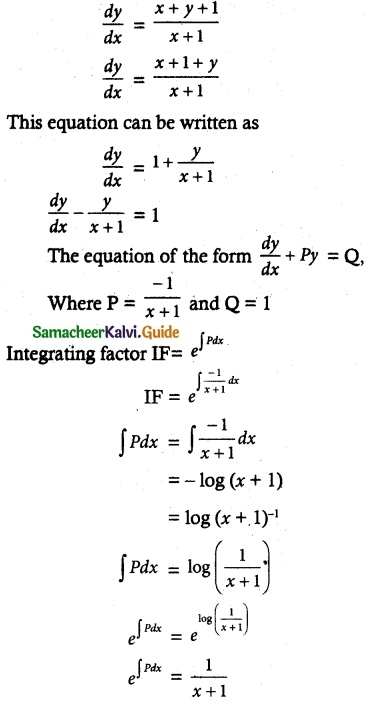Question 22.
The population P in any year t is such that the rate of increase in the population is proportional to the population. Then
(a) P = Cekt
(b) P = Ce-kt
(c) P = Ckt
(d) Pt = C
Solution:
(a) $$\frac { 1 }{ x+1 }$$
Hint: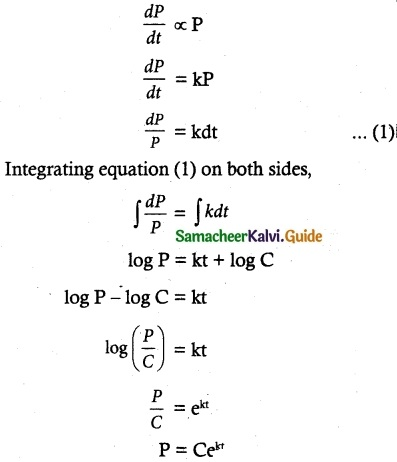Question 23.
P is the amount of certain substance left in after time t. If the rate of evaporation of the substance is proportional to the amount remaining, then
(a) P = Cekt
(b) P = Ce-kt
(c) P = Ckt
(d) Pt = C
Solution:
(b) P = Ce-kt
Hint: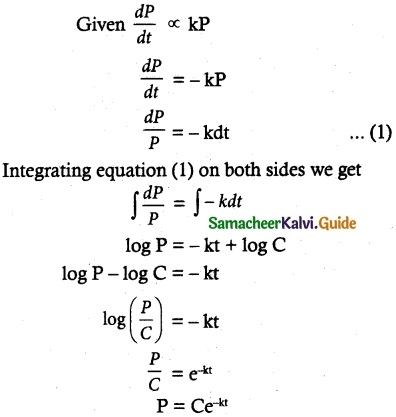Question 24.
If the solution of the differential equation $$\frac { dy }{ dx }$$ = $$\frac { ax+3 }{ 2y+f }$$ represents a circle, then the value of a is
(a) 2
(b) -2
(c) 1
(d) -1
Solution:
(b) -2
Hint:
Given $$\frac { dy }{ dx }$$ = $$\frac { ax+3 }{ 2y+f }$$
The equation can be written as
(2y + f) dy = (ax + 3) dx ……. (1)
Integrating equation (1) on both sides, we get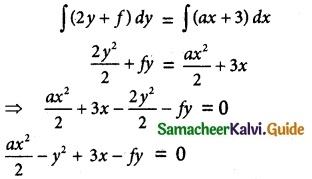It is solution of the given differential equation.
Since this solution represents a circle,
co-efficient of x² = co-efficient of y²
i.e) $$\frac { a }{ 2 }$$ = -1
a = -2

Question 25.
The slope at any point of a curve y = f(x) is given by $$\frac { dy }{ dx }$$ = 3x² and it passes through (-1, 1). Then the equation of the curve is
(a) y = x³ + 2
(b) y = 3x² + 4
(c) y = 3x³ + 4
(d) y = x³ + 5
Solution:
(a) y = x³ + 2
Hint:
Given differential equation is $$\frac { dy }{ dx }$$ = 3x²
The equation can be written as dy = 3x² dx ……… (1)
Integrating equation (1) on both sides, we get
∫dy = ∫ 3x²dx
y = $$\frac { 3x^3 }{ 3 }$$ + C
y = x³ + C …….. (2)
Since it passes through (-1, 1)
So, y = x³ + C becomes
1 =(-1)³ + C
1 = -1 + C
1 + 1 = C
∴ C = 2
Substituting C value in equation (2), We get The equation of the curve is y = x³ + 2